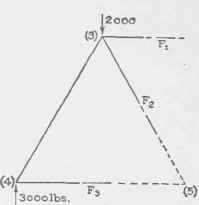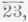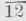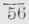Hence, assuming F1' to be a pull,

-(F1" X 7)+ (3,000 X 7)+ (3,000 X 17.5) +(1,500 X 28) - (6,000

X 28) = 0, or F1 =[ (3,000 X 7)+ (3,000 X 17.5) +(1,500 X 28) -(6,000 X 28)] / 7

= - 7,500

The minus sign means that F1' is a push, hence the memberis under compression of 7,500 pounds, a result agreeing with that previously found.

2. It is required to find the stress in the member gh of the truss represented in Fig. 25, due to the loads shown.

If we pass a section cutting bg, gh and he, and consider the left part, we get Fig. 40, the forces on that part being the 2,000-pound load, the left reaction, and the forces F1, F2 and F3 exerted by the right part on the left. To compute F2 it is simplest to use the condition that the algebraic sum of all the vertical components equals zero. Thus, assuming that F2 is a pull, and since its angle with the vertical is 30°,

F2 cos 30°-2,000 + 3,000 = 0; or,

F2= 2,000-3,000 /cos 30° = -1,000 /0.866 =-1,154.

The minus sign means that F2 is a push, hence the member is under a compression of 1,154 pounds. 3. It is required to determine the stress in the member bg of the truss represented in Fig. 25, due to the loads shown.

If we pass a section cutting bg as in the preceding illustra-tion, and consider the left part, we get Fig. 40. To compute F1 it is simplest to write the moment equation for all the forces using joint 5 as origin. From a large scale drawing, we measure the arm of F1 to be 13.86 feet hence, assuming F1 to be a pull,

F1X 13.86-2,000 X 8 + 3,000 X 16 = 0; or

F1= [2,000 X 8-3,000 X 16]/13.86=-32,000/13.86

= - 2,309.

The minus sign means that F1 is a push; hence the member is under a compression of 2,308 pounds.

The section might have been passed so as to cut members fg, fg, and fe, giving Fig. 41 as the left part, and the desired force might be obtained from the system of forces acting on that part (3,000, 2,000, F1, F2, and F3.) To compute F1 we take moments about the intersection of F2 and F3, thusFig. 41.Fig. 42.

F1 X 13.86 - 2,000 X 8 + 3,000 X 16 = 0.

This is the same equation as was obtained in the first solution, and hence leads to the same result.

4. It is required to determine the stress in the memberof the truss represented in Fig. 26, due to the loads shown.

Passing a section cutting; members and considering the left part, we get Fig. 42. To determine Ft we may write a moment equation preferably with origin at joint 4, thus:andF1 X 4.47 + 4,000 X 10 = 0*; or, F1=-4,000x10/4.47=-8,948 pounds, the minus sign meaning that the stress is compressive.

F1might be determined also by writing the algebraic sum of the vertical components of all the forces on the left part equal to zero, thus:Fig. 43.

F1 sin 26° 34' + 4,000 = 0; or, F1= - 4,000/sin 260 34' =- 4,000/0.447= - 8,948, agreeing with the first result.

## Examples For Practice

1. Determine by the method of sections the stresses in members of the truss represented in Fig. 26 due to the loads shown.andAns. Stress in 23 Stress in 25 = - 5,000 pounds, = - 3,350 pounds. Stress in 45 = + 8,000 pounds

* The arm of F1with respect to (4) is 4.47 feet.

2. Determine the stresses in the members of the truss represented in Fig. 27, due to the loads shown.andAns. Stress in= -- 11,170 pounds, Stress in= + 10,000 pounds, Stress in= - 8,940 pounds, Stress in= + 6,000 pounds.Fig. 44.

40. Complete Analysis of a Fink Truss. As a final illustration of analysis, we shall determine the stresses in the members of the truss represented in Fig. 43, due to permanent, snow, and wind loads. This is a very common type of truss, and is usually called a "Fink" or "French" truss. The trusses are assumed to be 15 feet apart; and the roof covering, including purlins, such that it weighs 12 pounds per square foot.

The length from one end to the peak of the truss equals" = 33.54 feet, hence the area of the roofing sustained by one truss equals

(33.54 X 15) 2 = 1,006.2 square feet, and the weight of that portion of the roof equals 1,006.2 X 12 = 12,074 pounds.UNIVERSITY HALL, MAIN BUILDING OF WASHINGTON UNIVERSITY, ST. LOUIS, MO.

Cope & Stewardson, Architects, Philadelphia and St. Louis.

This Building, the Gift of Mr. Robert S. Brookings, was Erected at a Cost of \$220,000. It Faces the Main Approach to the Group of New Buildings Adorning the University Campus. The Main Portion of the Structure is 261 Feet Long and 51 Feet Wide; the Two Wings. Each 119 Feet Long by 32 Feet Wide; and the Towers. 85 Feet High.

The probable weight of the truss (steel), according to the formula of Art. 19, is

15 X 60 (60/25+ 1)= 3,060 pounds.

The total permanent load, therefore, equals

12,074 + 3,060 = 15,131 pounds; the end loads equal 1/16 he total, or 950 pounds, and the other apex loads equal 1/8 the total, or 1,900 pounds.

Dead Load 5tress. To determine the dead load stresses, construct a stress diagram. Evidently each reaction equals one-half the total load, that is 7,600 pounds; therefore ABCDEFGHIJKA (Fig. 44b) is a polygon for all the loads and reactions. First, we draw the polygon for joint 1; it is KABLK, BL and LK representing the stress in bl and Ik (see record Page 72 for values). Next draw the polygon for joint 2; it is LBCML, CM and ML representing the stresses in cm and ml. Next draw the polygon for joint 3; it is KLMNK, MN and NK representing the stresses in mn and nk.